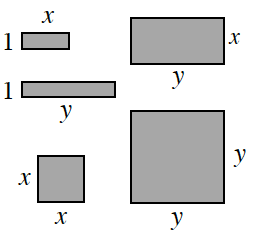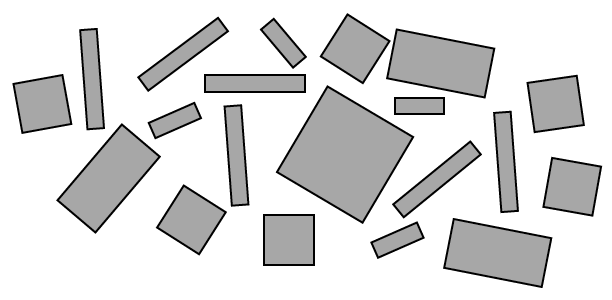### Home > CCA > Chapter A > Lesson A.1.2 > ProblemA-21

A-21.

If algebra tiles have the dimensions shown at right, what would you call the tile collection below? (What is the total area of all of the pieces?) Write the expression algebraically, using $x, x^{2}, y, y^{2}$, and $xy$.To solve this problem, you will need to group the shapes by their area.
How many $x$-tiles are there? $x^{2}$ -tiles? Count the number of each different area.

$6x^{2} + 4x + 3xy + 6y + y^{2}$

Use the eTool below to solve the problem.
Click the link at right for the full version of the eTool: CCA A-21 HW eTool